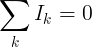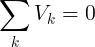# 基爾霍夫定律

## 基爾霍夫現行法律（KCL）#### KCL示例

12進入路口

3離開路口

I 1 = 2A，I 2 = 3A，I 3 = -1A，I 4 =？

I k = I 1 + I 2 + I 3 + I 4 = 0

I 4 = -I 1 -I 2 -I 3 = -2A-3A-（-1A）= -4A

## 基爾霍夫電壓定律（KVL）#### KVL示例

V S = 12V，V R1 = -4V，V R2 = -3V

V R3 =？

V k = V S + V R1 + V R2 + V R3 = 0

V R3 = - V小號- V R1 - V R2 = -12V + 4V + 3V = -5V